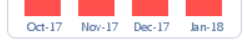# New to QlikView

Discussion board where members can get started with QlikView.

Announcements
Make your voice heard! Participate in the 2020 Wisdom of Crowds® Survey. BEGIN SURVEY
Highlighted
New Contributor III

## How do you calculate STDEV of past four dates, using totals, instead of individual values?

Hi -

I am trying to calculate the Standard Deviation of the last four dates to color each bar a different color. Whenever I try to calculate the Standard Deviation of all four dates, Qlik keeps on calculating as if I am looking at individual field values (each date is powered by several hundred entries).

I am simply trying to calculate the standard deviation of the numbers at the top of the bar chart: 45.32, 27.06, 41,26, and 52.57.

The field name powering the totals is "Sum_Total", and the date field is "Date".

When I try = STDEV({<Date=>}Sum_Total), thinking that I would be able to disregard every date field, I get a very low number.

Any way to group the sum of each date and then calculate the standard deviation separately? I want to be able to power the color of each bar chart based on if it is a certain number of units above the standard deviation, below, etc.

Thanks!

Ricky

1 Solution

Accepted Solutions
HighlightedMVP

## Re: How do you calculate STDEV of past four dates, using totals, instead of individual values?

Use this

Date(MonthStart(Raw_Date), 'MMM-YY')) as MonthYear

and now use the expression

Stdev({<Date>} Aggr(Sum({<Date>} Sum_Total), MonthYear))

7 Replies
HighlightedMVP

## Re: How do you calculate STDEV of past four dates, using totals, instead of individual values?

May be like this

Stdev({<Date>} Aggr(Sum({<Date>} Sum_Total), MonthYear))

Highlighted
New Contributor III

## Re: How do you calculate STDEV of past four dates, using totals, instead of individual values?

I just tried Sunny, thanks. It doesn't work - what does the "MonthYear" stand for, is that a calculated field?

HighlightedMVP

## Re: How do you calculate STDEV of past four dates, using totals, instead of individual values?

The dimension of your chart... I hope it is not a calculated dimensions.... because if it is, then I would suggest you to create a field in the script and use that in the Aggr() functionHighlighted
New Contributor III

## Re: How do you calculate STDEV of past four dates, using totals, instead of individual values?

It is a calculated field, which I wrote in the back-end:

date(Raw_Date,'MMM-YY') as Date

How to I add AGGR to this?

HighlightedMVP

## Re: How do you calculate STDEV of past four dates, using totals, instead of individual values?

Use this

Date(MonthStart(Raw_Date), 'MMM-YY')) as MonthYear

and now use the expression

Stdev({<Date>} Aggr(Sum({<Date>} Sum_Total), MonthYear))

Highlighted
New Contributor III

## Re: How do you calculate STDEV of past four dates, using totals, instead of individual values?

awesome - I think it works!!

HighlightedMVP

## Re: How do you calculate STDEV of past four dates, using totals, instead of individual values?

Nice